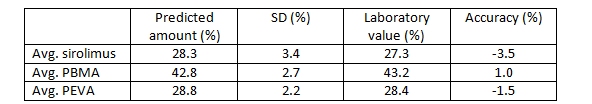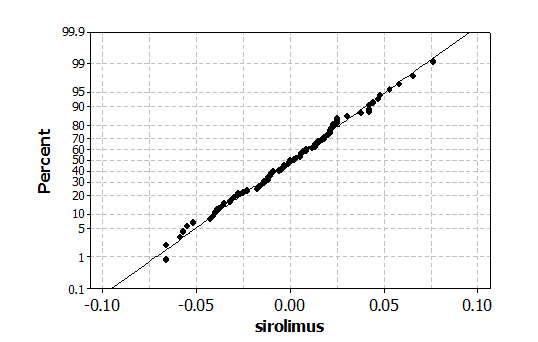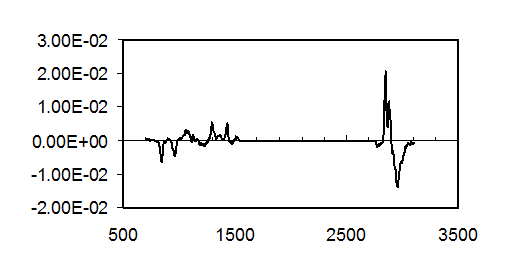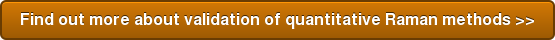# Spectroscopy Solutions Blog

This article is the second of a three part series on quantitative prediction using Raman spectroscopy. Method validation is the process of verifying an analytical method is performing as required. Method validation is required in regulated industries and is good scientific practice. United States Pharmacopeia chapters on Near IR spectroscopy and on Raman spectroscopy describe the criteria that should be used to validate a quantitative method using NIR or Raman spectroscopy. The criteria are accuracy, precision, linearity, range, robustness and specificity.

Accuracy describes how close the predicted values are to the actual laboratory reference values.  Precision defines how reproducible predictions are either in consecutive measurements or when different analysts perform the measurements. In validation of any chemometric method, the use of independent samples is essential. Testing with independent samples eliminates the possibility of overfitting the calibration set data. Furthermore the independent samples should challenge the model in question by presenting real-world variation to the model. The accuracy of the PLS models for sirolimus, PEVA and PBMA were tested with a set of independent production samples. The results are shown in Table 1.Table 1: Validation results for PLS methods. Note the laboratory value of sirolimus was found by HPLC and the polymer values were by gravimetric methods.

Accuracy as expressed as a percent different between the lab and the PLS prediction values was less than 4% for all three components. The standard deviation (SD) values in Table 1 are a measure of the precision of the drug and polymer PLS methods. We note that the PLS models were found to be more accurate than the simpler univariate models. This was even true in the cases of sirolimus, where a very strong peak associated with drug could be used for the univariate calibration. The sum of all predictions for the drug, PEVA and PBMA ranged from 99.1 to 100.8%. This result demonstrates that PLS methods also exhibited mass balance.

The linearity of the chemometric model can be evaluated by an examination of the residuals for the calibration curves or a validated set of data, if available. Residuals are the difference between the PLS prediction and actual lab values for a specific sample of interest. A linear model will exhibit a random or normally distributed set of residuals. Visual inspection of the set of residuals can be used to look for obvious non-linearity in the regression model. A simple way to examine a set of data for normality is a normal probability plot. A normal probability plot of the residuals for the PLS sirolimus calibration is shown in Figure 1. The actual residual values are plotted on the x-axis, and an estimated cumulative probability is plotted on the y-axis. The cumulative probability is the probability that a measurement is less than or equal to its x-axis value. Therefore, in Figure 1, the larger magnitude positive residuals have a cumulative probability close to 1.0. The visual interpretation of the normal probability plot is straightforward.  If the points in the normal probability plot follow a straight line, then the data is normally distributed. Examination of the data in Figure 1 demonstrates the linearity of the model.Figure 1: Normal probability plot of the residuals for the sirolimus calibration curve.

The specificity of a PLS method is documented by examination of the regression vector. The regression vector is a summary of a PLS method for a given number of factors or principal components. Raman shift values with a large positive or negative regression coefficient value are important for the prediction of the constituent of interest. These peaks should correspond to the actual experimental peaks in the measured Raman spectrum of the compound, whose concentration is being predicted. Examination of the regression coefficient for either polymer is illustrative. The regression coefficient for PEVA is shown in Figure 2. The peaks in the regression coefficient are experimental spectrum of PEVA shown in the previous article. This includes the two peaks at Raman shifts less than 1000 cm-1.Figure 2: Regression coefficient for PEVA.The x-axis is Raman shift in units of (cm-1).

The range of a chemometric method is determined by the range of the calibration samples.  The range of values for the polymer and drug models is described in the previous article. The robustness of the PLS methods was maintained by using models with only  a modest number of factors. Robustness of a model based on Raman spectroscopy can also be tested by changing the sample position for a homogenous sample or by making a small change to the laser intensity.

In summary this article discusses the validation of PLS methods for drug, PEVA and PBMA as determined by Raman spectroscopy. The validation of any method is essential in order to understand the performance of the method.

The next article in this series will discuss quantitative predictions of drug and polymer spatial distributions using confocal Raman spectroscopy.

References
This article is a short summary of the following paper “Multivariate Analysis Applied to the Study of Spatial Distributions Found in Drug-Eluding Stent Coatings By Confocal Raman Microscopy”, K. Balss, F. Long, V. Veslov, E. Akerman, G. Papandreou, C. Maryanoff, Anal. Chem. 80 , 4853-4859 (2008).

United States Pharmacopeia chapters (1119) and (1120) Near IR spectroscopy and Raman spectroscopy respectively.Back to list